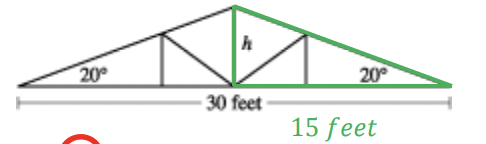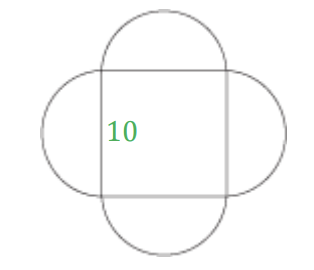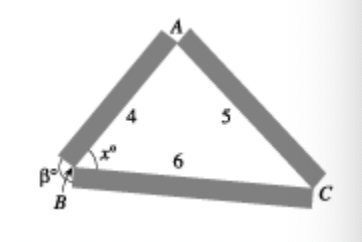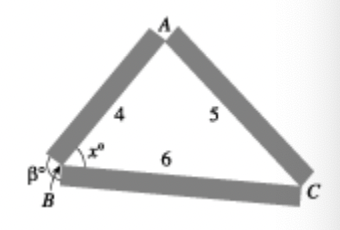# ACT Mathematics: Coordinate Geometry and Quadrilaterals Quiz

This is an MCQ-based quiz on the topic of ACT Mathematics: Coordinate Geometry and Quadrilaterals Quiz.

This set of MCQs helps you brush up on the important mathematics topic and prepare you to dive into skill practice and expand your knowledge to tackle the question and answer it carefully.

Start Quiz

What is the x‐intercept of the graph of y = x^2 – 4x + 4 ?

-2

-1

0

2

Which of the following expressions is the closest approximation to the height h, in feet, of the roof truss shown below?15

15 tan20

15 sin20

15 cos20

Which of the following expressions is the closest approximation to the height h, in feet, of the roof truss shown below?15

15 tan20

15 sin20

15 cos20

The geometric figure shown below consists of a square and 4 semicircles. The diameters of the semicircles are the sides of the square, and each diameter is 10 centimeters long. Which of the following is the closest approximation of the total area, in square centimeters, of this geometric figure?100

160

260

400

A neighborhood recreation program serves a total of 280 children who are either 11 years old or 12 years old. The sum of the children’s ages is 3,238 years. How many 11‐year‐old children does the recreation program serve?

55

122

132

158

Which one of the following expressions has an even integer value for all integers a and c ?

8a + 2ac

3a + 3c

2a + c

a + 2c

After arranging the flower bed, Taher decides that the flower bed would look more attractive if 1 of the angles in the triangle were a right angle. He decides to place the right angle at vertex A and to leave the lengths of AB and AC as 4 and 5 feet, respectively. To the nearest 0.1 foot, how long of a piece of lumber would he need to replace the 6‐foot piece represented by BC ?

3

3.3

6

6.4

Use the following information to answer questions 3–5. Taher has decided to create a triangular flower bed border. He plans to use 3 pieces of rectangular lumber with lengths 4, 5, and 6 feet, as shown in the figure below. Points A, B, and C are located at the corners of the flower bed.The measure of <ABC in the figure is x°. Which of the following is an expression for b° ?

x

2x

90 + x

180 -x

Use the following information to answer questions 3–5. Taher has decided to create a triangular flower bed border. He plans to use 3 pieces of rectangular lumber with lengths 4, 5, and 6 feet, as shown in the figure below. Points A, B, and C are located at the corners of the flower bed.

Taher plans to cut the 3 pieces of lumber for the flower bed border from a single piece of lumber. Each cut takes ଵ ଼ inch of wood off the length of the piece of lumber. Among the following lengths, in inches, of pieces of lumber, which is the shortest piece that he can use to cut the pieces for the flower bed border?178

179

180

181

Which of the following is an equation of the circle with its center at (0,0) that passes through (3,4) in the standard (x,y) coordinate plane?

x-y =1

x+y = 25

x^2 + y = 25

x^2 + y^2 = 25

Quiz/Test Summary
Title: ACT Mathematics: Coordinate Geometry and Quadrilaterals Quiz
Questions: 10
Contributed by: